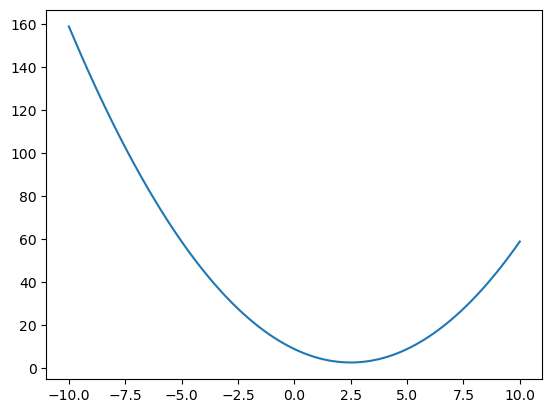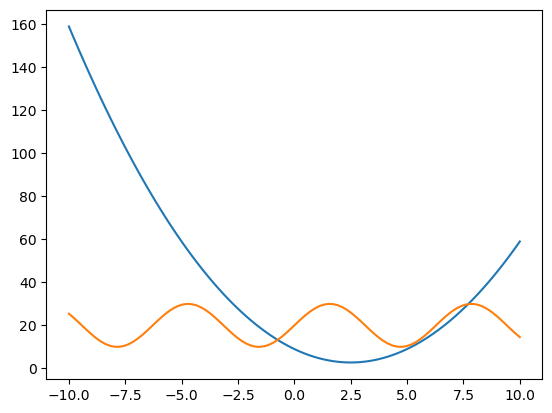# How to graph mathematical functions (in Python, using NumPy and Matplotlib)

See all solutions.

Assume we have a mathematical formula and we would like to plot a graph of it using the standard Cartesian coordinate system.

## Solution

Let’s assume we want to graph the function $x^2-5x+9$ from $x=-10$ to $x=10$. Let’s import NumPy for the mathematics and Matplotlib for the graph.

1
2
import numpy as np
import matplotlib.pyplot as plt


We compute a series of $(x,y)$ pairs to generate the plot. Notice how NumPy automatically computes a $y$ value for each $x$ value if we just include all the $x$s in the formula we wish to graph.

1
2
3
4
xs = np.linspace( -10, 10, 100 )   # 100 values from x=-10 to x=10
ys = xs**2 - 5*xs + 9              # compute all corresponding ys
plt.plot( xs, ys )
plt.show()You can also plot more than one function on the same graph.

1
2
3
4
ys2 = 10*np.sin(xs) + 20          # ys for the formula y=10sin(x)+20
plt.plot( xs, ys )                # make the original plot
plt.plot( xs, ys2 )               # add the second plot to it
plt.show()